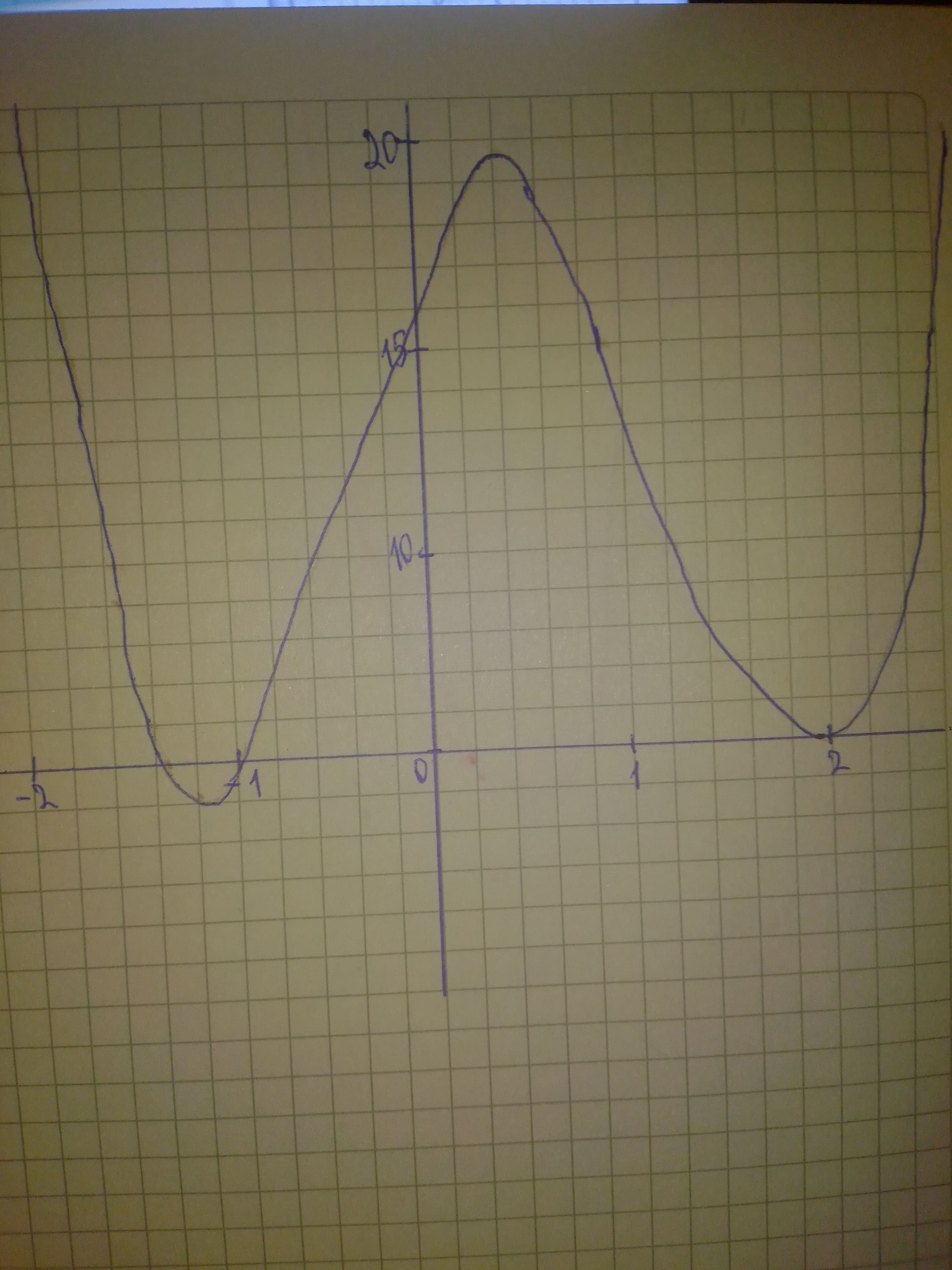Wotzdorfg

2020-10-21

Solve the polynomial inequality graphically. $3{x}^{4}-5{x}^{3}-12{x}^{2}+12x+16$Sally Cresswell

Step 1
We graph the left-hand side of the inequality and find x-intercepts at $x=-\frac{4}{3},x=-1,x=2$:Do you have a similar question?